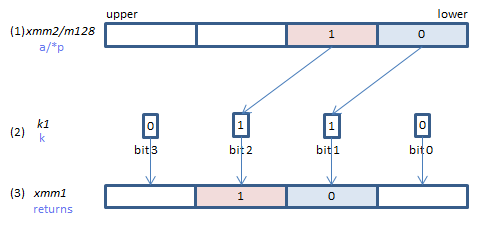﻿ vexpandps

## VEXPANDPS - EXPAND Packed Single

VEXPANDPS xmm1{k1}{z}, xmm2/m128    (V5+VLIf each bit of (2) is set, the corresponding element of (3) is copied from (1), taken one by one from the lowest element.
If corresponding bit of (2) is not set, (3) element is:
zero cleared if {z} is specified (_maskz_ intrinsic is used)
left unchanged if {z} is not specified. (copied from s if _mask_ intrinsic is used.)
VEXPANDPS ymm1{k1}{z}, ymm2/m256    (V5+VLIf each bit of (2) is set, the corresponding element of (3) is copied from (1), taken one by one from the lowest element.
If corresponding bit of (2) is not set, (3) element is:
zero cleared if {z} is specified (_maskz_ intrinsic is used)
left unchanged if {z} is not specified. (copied from s if _mask_ intrinsic is used.)
VEXPANDPS zmm1{k1}{z}, zmm2/m512    (V5